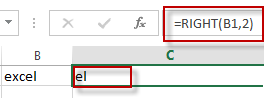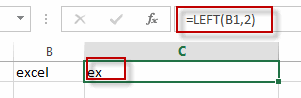# Extract First or Last N Characters

This post will guide you how to extract the first or last n characters from a text string in one cell in Excel. How do I get the first or last N characters from a string using an Excel formula. How to extract first two or last two characters of a cell with a formula in Excel.

## Extract First N Characters

If you want to extract the first n characters of a sting in a cell in Excel, you can use the LEFT function to create a formula to achieve it. For example, you want to extract the first two characters from a text string in Cell B1, just using the following formula:

`=LEFT(B1,2)`Type this formula into a blank cell, and then press Enter key in your keyboard.

The First two characters are extracted in Cell C1.

## Extract Last N Characters

To extract the last N characters in a cell in excel, you need to use the RIGHT function to achieve it. For examples, you want to extract the last two characters from a text string in cell B1, just using the below formula:

`=RIGHT(B1,2)`Type this formula into a blank cell, and then press Enter key.

The last two characters are extracted in Cell C1.

### Related Functions

• Excel LEFT function
The Excel LEFT function returns a substring (a specified number of the characters) from a text string, starting from the leftmost character.The LEFT function is a build-in function in Microsoft Excel and it is categorized as a Text Function.The syntax of the LEFT function is as below:= LEFT(text,[num_chars])…
• Excel RIGHT function
The Excel RIGHT function returns a substring (a specified number of the characters) from a text string, starting from the rightmost character.The syntax of the RIGHT function is as below:= RIGHT (text,[num_chars])…